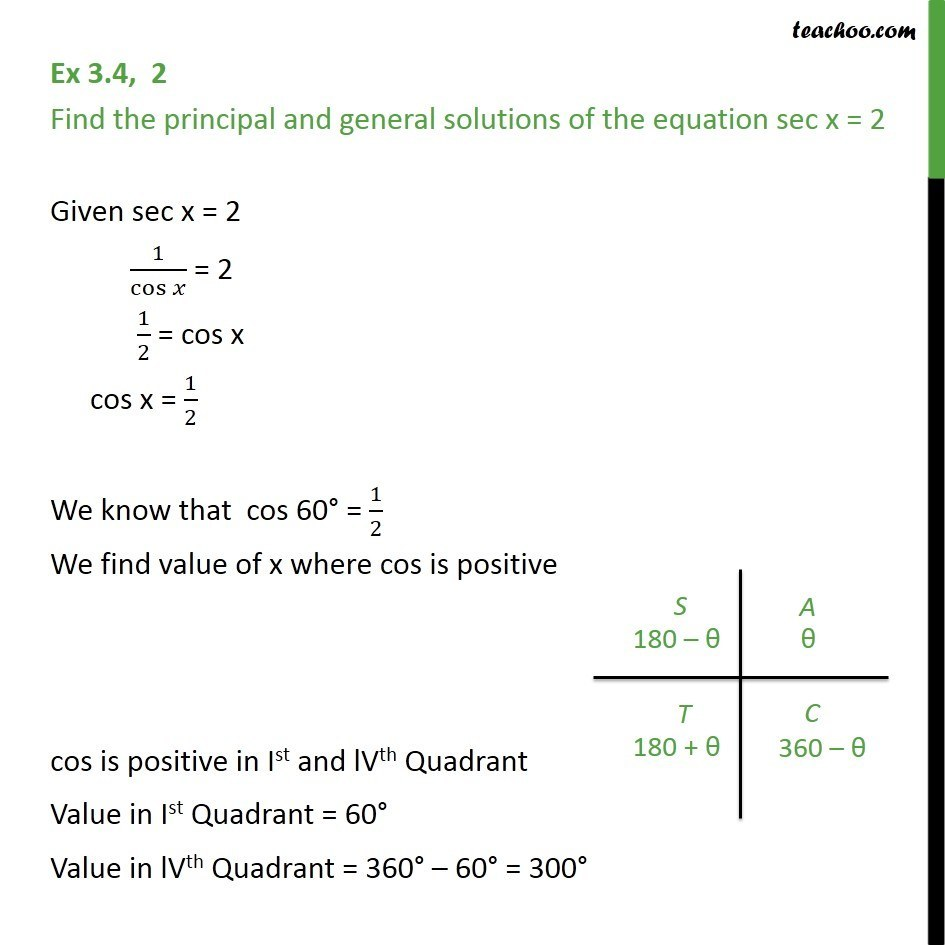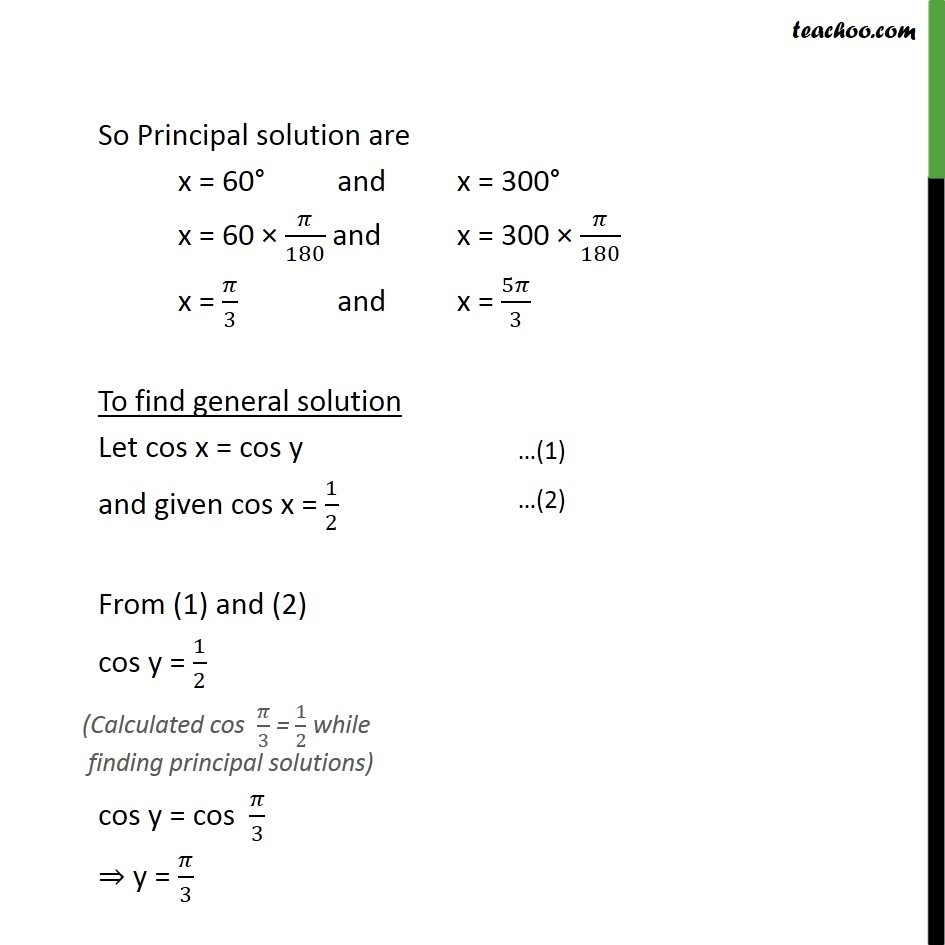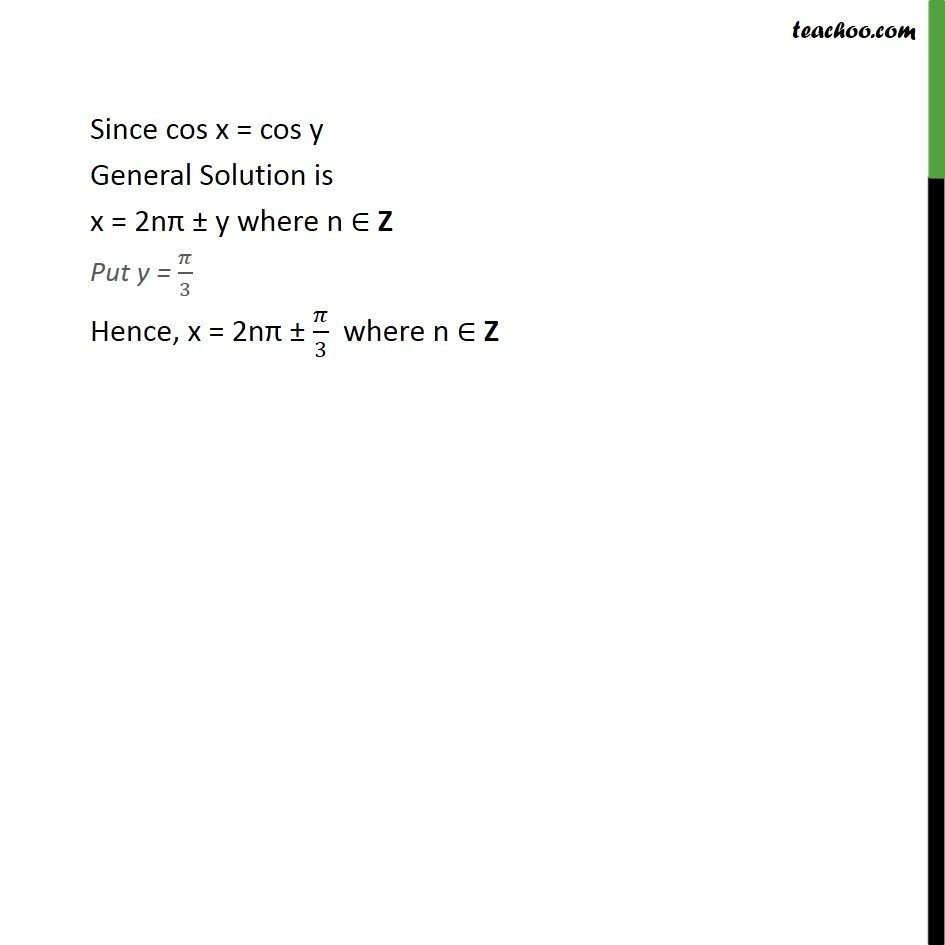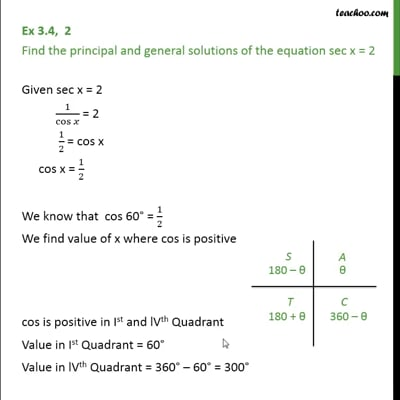Ex 3.4

Chapter 3 Class 11 Trigonometric Functions (Term 2)
Serial order wiseThis video is only available for Teachoo black users

### Transcript

Ex 3.4, 2 Find the principal and general solutions of the equation sec x = 2 Given sec x = 2 1/cos⁡𝑥 = 2 1/2 = cos x cos x = 1/2 We know that cos 60° = 1/2 We find value of x where cos is positive cos is positive in Ist and lVth Quadrant Value in Ist Quadrant = 60° Value in lVth Quadrant = 360° – 60° = 300° So Principal solution are x = 60° and x = 300° x = 60 × 𝜋/180 and x = 300 × 𝜋/180 x = 𝜋/3 and x = 5𝜋/3 To find general solution Let cos x = cos y and given cos x = 1/2 From (1) and (2) cos y = 1/2 cos y = cos 𝜋/3 ⇒ y = 𝜋/3 Since cos x = cos y General Solution is x = 2nπ ± y where n ∈ Z Put y = 𝜋/3 Hence, x = 2nπ ± 𝜋/3 where n ∈ Z